# 2.7 Linear inequalities and absolute value inequalities  (Page 6/11)

 Page 6 / 11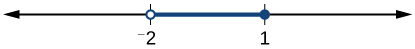$\left(-2,1\right]$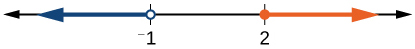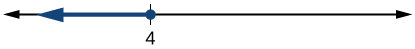$\left(-\infty ,4\right]$

## Technology

For the following exercises, input the left-hand side of the inequality as a Y1 graph in your graphing utility. Enter y2 = the right-hand side. Entering the absolute value of an expression is found in the MATH menu, Num, 1:abs(. Find the points of intersection, recall (2 nd CALC 5:intersection, 1 st curve, enter, 2 nd curve, enter, guess, enter). Copy a sketch of the graph and shade the x -axis for your solution set to the inequality. Write final answers in interval notation.

$|x+2|-5<2$

$\frac{-1}{2}|x+2|<4$

Where the blue is below the orange; always. All real numbers. $\text{\hspace{0.17em}}\left(-\infty ,+\infty \right).$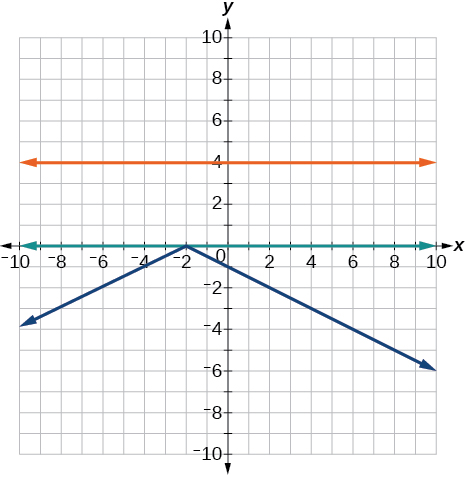$|4x+1|-3>2$

$|x-4|<3$

Where the blue is below the orange; $\text{\hspace{0.17em}}\left(1,7\right).$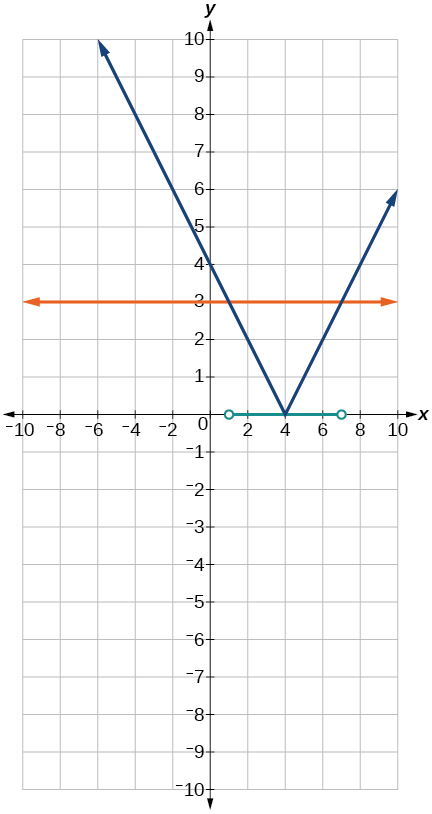$|x+2|\ge 5$

## Extensions

Solve $\text{\hspace{0.17em}}|3x+1|=|2x+3|$

$x=2,\frac{-4}{5}$

Solve ${x}^{2}-x>12$

$\frac{x-5}{x+7}\le 0,$ $x\ne -7$

$\left(-7,5\right]$

$p=-{x}^{2}+130x-3000\text{\hspace{0.17em}}$ is a profit formula for a small business. Find the set of x -values that will keep this profit positive.

## Real-world applications

In chemistry the volume for a certain gas is given by $\text{\hspace{0.17em}}V=20T,$ where V is measured in cc and T is temperature in ºC. If the temperature varies between 80ºC and 120ºC, find the set of volume values.

$\begin{array}{l}80\le T\le 120\\ 1,600\le 20T\le 2,400\end{array}$

A basic cellular package costs $20/mo. for 60 min of calling, with an additional charge of$.30/min beyond that time.. The cost formula would be $\text{\hspace{0.17em}}C=\text{}20+.30\left(x-60\right).\text{\hspace{0.17em}}$ If you have to keep your bill lower than \$50, what is the maximum calling minutes you can use?

## The Rectangular Coordinate Systems and Graphs

For the following exercises, find the x -intercept and the y -intercept without graphing.

$4x-3y=12$

x -intercept: $\text{\hspace{0.17em}}\left(3,0\right);$ y -intercept: $\text{\hspace{0.17em}}\left(0,-4\right)$

$2y-4=3x$

For the following exercises, solve for y in terms of x , putting the equation in slope–intercept form.

$5x=3y-12$

$y=\frac{5}{3}x+4$

$2x-5y=7$

For the following exercises, find the distance between the two points.

$\left(-2,5\right)\left(4,-1\right)$

$\sqrt{72}=6\sqrt{2}$

$\left(-12,-3\right)\left(-1,5\right)$

Find the distance between the two points $\text{\hspace{0.17em}}\left(-71,432\right)\text{\hspace{0.17em}}$ and $\text{\hspace{0.17em}}\text{(511,218)}\text{\hspace{0.17em}}$ using your calculator, and round your answer to the nearest thousandth.

$620.097$

For the following exercises, find the coordinates of the midpoint of the line segment that joins the two given points.

midpoint is $\text{\hspace{0.17em}}\left(2,\frac{23}{2}\right)$

For the following exercises, construct a table and graph the equation by plotting at least three points.

$y=\frac{1}{2}x+4$

$4x-3y=6$

 x y 0 −2 3 2 6 6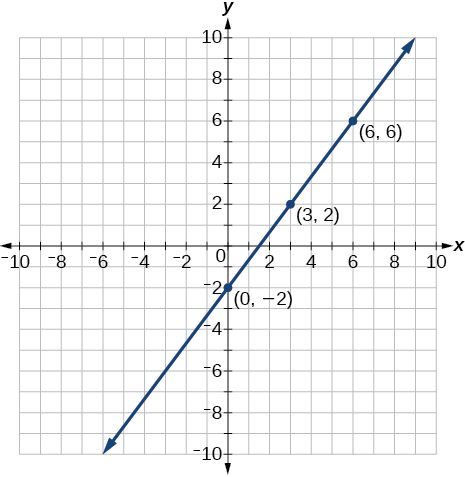## Linear Equations in One Variable

For the following exercises, solve for $\text{\hspace{0.17em}}x.$

$5x+2=7x-8$

$3\left(x+2\right)-10=x+4$

$x=4$

$7x-3=5$

$12-5\left(x+1\right)=2x-5$

$x=\frac{12}{7}$

$\frac{2x}{3}-\frac{3}{4}=\frac{x}{6}+\frac{21}{4}$

For the following exercises, solve for $\text{\hspace{0.17em}}x.\text{\hspace{0.17em}}$ State all x -values that are excluded from the solution set.

$\frac{x}{{x}^{2}-9}+\frac{4}{x+3}=\frac{3}{{x}^{2}-9}\text{\hspace{0.17em}}$ $x\ne 3,-3$

No solution

$\frac{1}{2}+\frac{2}{x}=\frac{3}{4}$

For the following exercises, find the equation of the line using the point-slope formula.

Passes through these two points: $\text{\hspace{0.17em}}\left(-2,1\right)\text{,}\left(4,2\right).$

$y=\frac{1}{6}x+\frac{4}{3}$

Passes through the point $\text{\hspace{0.17em}}\left(-3,4\right)\text{\hspace{0.17em}}$ and has a slope of $\text{\hspace{0.17em}}\frac{-1}{3}.$

Passes through the point $\text{\hspace{0.17em}}\left(-3,4\right)\text{\hspace{0.17em}}$ and is parallel to the graph $\text{\hspace{0.17em}}y=\frac{2}{3}x+5.$

$y=\frac{2}{3}x+6$

Passes through these two points: $\text{\hspace{0.17em}}\left(5,1\right)\text{,}\left(5,7\right).$

## Models and Applications

For the following exercises, write and solve an equation to answer each question.

The number of males in the classroom is five more than three times the number of females. If the total number of students is 73, how many of each gender are in the class?

females 17, males 56

#### Questions & Answers

A laser rangefinder is locked on a comet approaching Earth. The distance g(x), in kilometers, of the comet after x days, for x in the interval 0 to 30 days, is given by g(x)=250,000csc(π30x). Graph g(x) on the interval [0, 35]. Evaluate g(5)  and interpret the information. What is the minimum distance between the comet and Earth? When does this occur? To which constant in the equation does this correspond? Find and discuss the meaning of any vertical asymptotes.
Kaitlyn Reply
The sequence is {1,-1,1-1.....} has
amit Reply
circular region of radious
Kainat Reply
how can we solve this problem
Joel Reply
Sin(A+B) = sinBcosA+cosBsinA
Eseka Reply
Prove it
Eseka
Please prove it
Eseka
hi
Joel
June needs 45 gallons of punch. 2 different coolers. Bigger cooler is 5 times as large as smaller cooler. How many gallons in each cooler?
Arleathia Reply
7.5 and 37.5
Nando
find the sum of 28th term of the AP 3+10+17+---------
Prince Reply
I think you should say "28 terms" instead of "28th term"
Vedant
the 28th term is 175
Nando
192
Kenneth
if sequence sn is a such that sn>0 for all n and lim sn=0than prove that lim (s1 s2............ sn) ke hole power n =n
SANDESH Reply
write down the polynomial function with root 1/3,2,-3 with solution
Gift Reply
if A and B are subspaces of V prove that (A+B)/B=A/(A-B)
Pream Reply
write down the value of each of the following in surd form a)cos(-65°) b)sin(-180°)c)tan(225°)d)tan(135°)
Oroke Reply
Prove that (sinA/1-cosA - 1-cosA/sinA) (cosA/1-sinA - 1-sinA/cosA) = 4
kiruba Reply
what is the answer to dividing negative index
Morosi Reply
In a triangle ABC prove that. (b+c)cosA+(c+a)cosB+(a+b)cisC=a+b+c.
Shivam Reply
give me the waec 2019 questions
Aaron Reply

### Read also:

#### Get the best Algebra and trigonometry course in your pocket!

Source:  OpenStax, Algebra and trigonometry. OpenStax CNX. Nov 14, 2016 Download for free at https://legacy.cnx.org/content/col11758/1.6
Google Play and the Google Play logo are trademarks of Google Inc.

Notification Switch

Would you like to follow the 'Algebra and trigonometry' conversation and receive update notifications?ByByBy Lakeima RobertsBy Anonymous UserBy RhodesBy Abishek DevarajBy Edward Biton